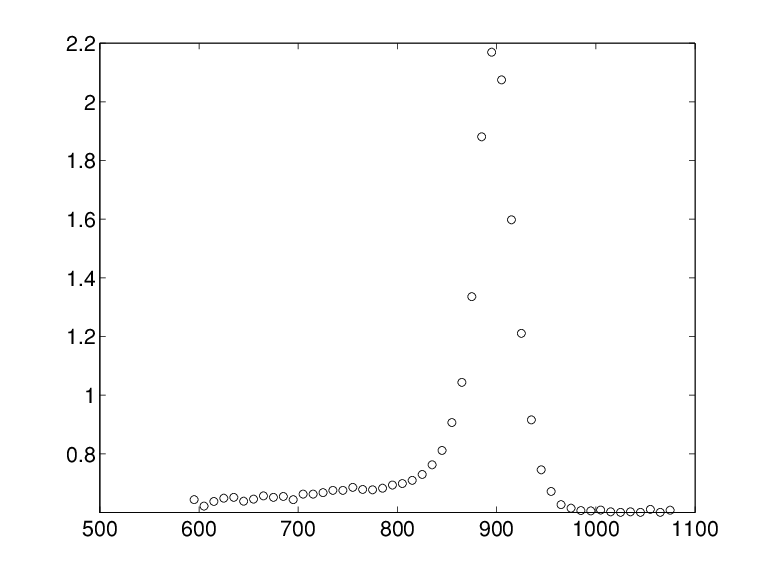Main Content

# titanium

Titanium test data

## Syntax

```[x,y] = titanium ```

## Description

`[x,y] = titanium ` returns measurements of a certain property of titanium as a function of temperature. Since their use in , these data have become a standard test for data fitting since they are hard to fit by classical techniques and have a significant amount of noise.

## Examples

The plot of the data shown below is generated by the following commands:

```[x,y] = titanium; plot(x,y,'ok'), set(gca,'Fontsize',16) ```C. de Boor and J. R. Rice. "Least squares cubic spline approximation II - Variable knots." CSD TR 21, Comp.Sci., Purdue Univ., April 1968.

## Support

#### Machine Learning Challenges: Choosing the Best Classification Model and Avoiding Overfitting

Download white paper